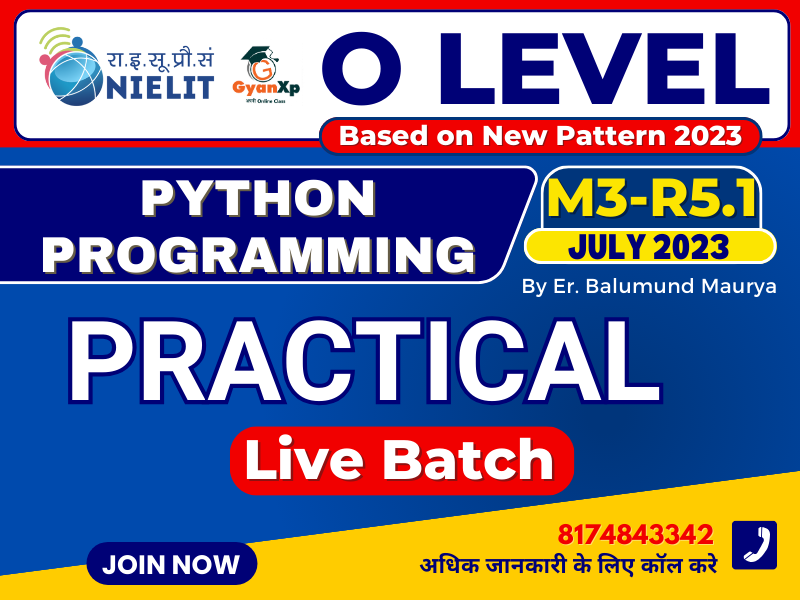## O Level Python Programming (M3-R5.1) Practical Demo

Q2. Write a Python function to find the sum of all numbers between 100 and 500 which are divisible by 2.

Q1. Write a program to print multiplication table of a given number

## O Level Practical की बेहरत तैयारी के लिए Join करे Practical Batch